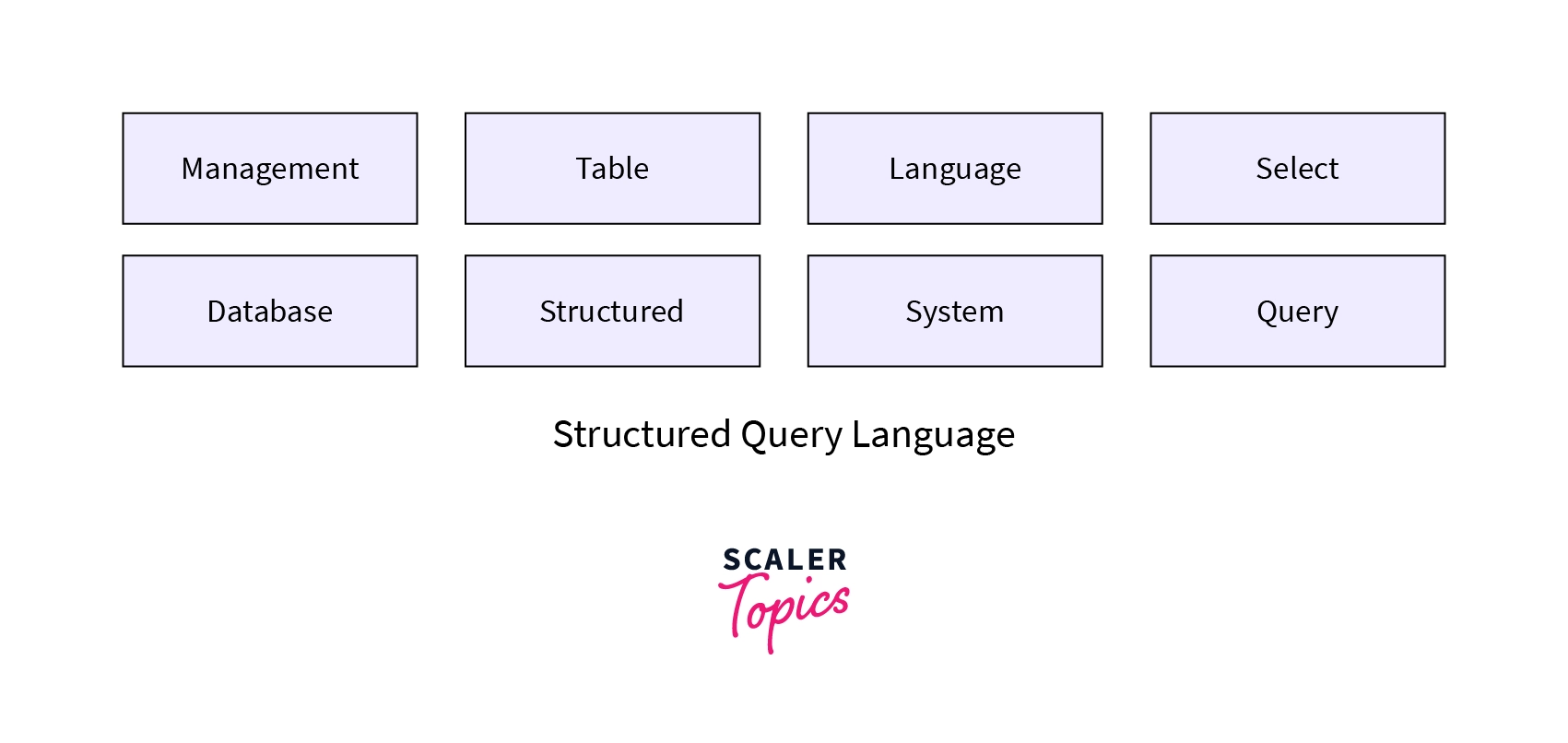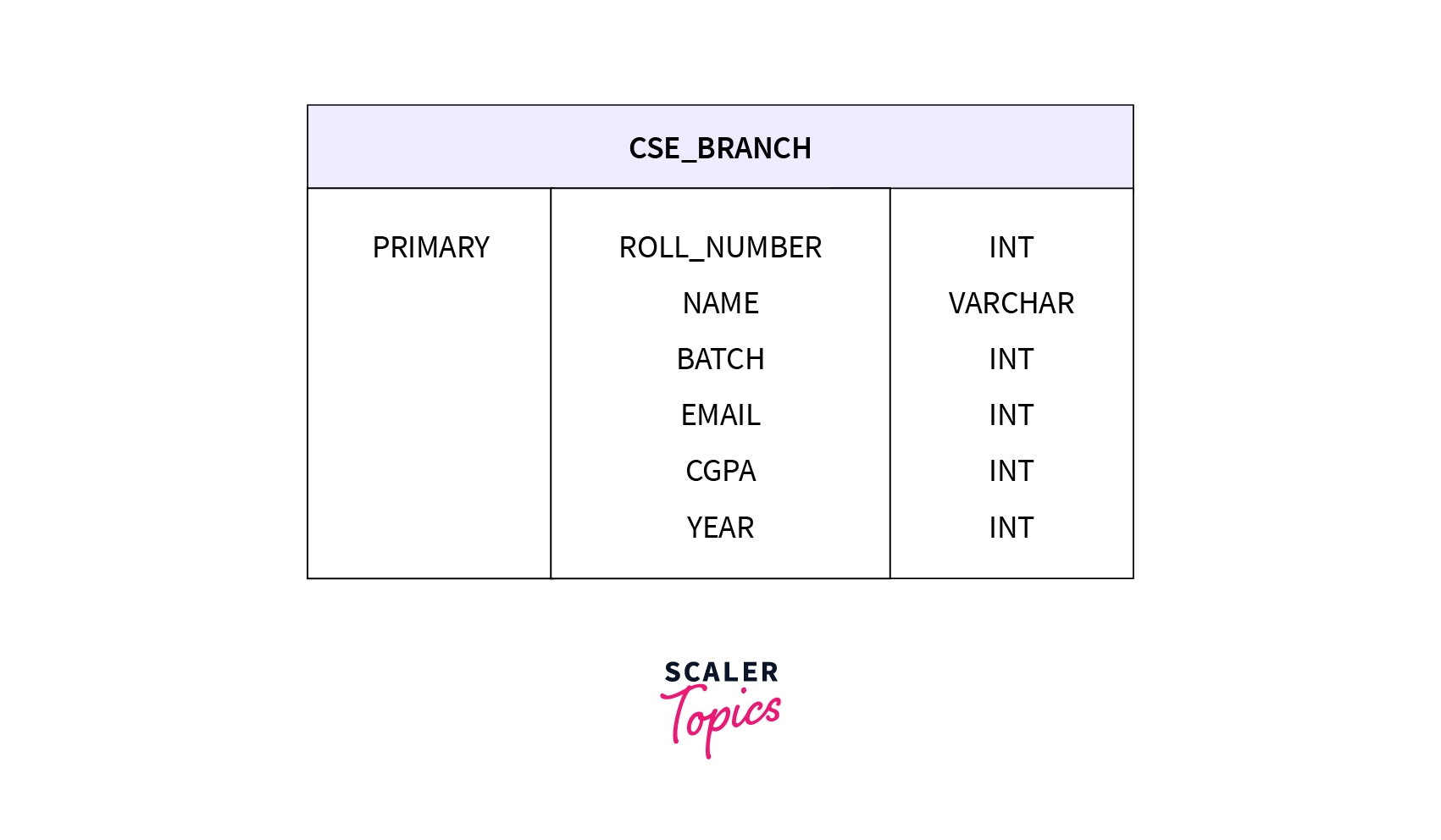# Keywords in SQL

Challenge Inside! : Find out where you stand! Try quiz, solve problems & win rewards!
Learn via video courseDBMS Course - Master the Fundamentals and Advanced Concepts
By Srikanth VarmaFree
5Enrolled: 1000DBMS Course - Master the Fundamentals and Advanced Concepts
Srikanth VarmaFree
5
Enrolled: 1000

## Overview

Keywords refer to the reserved set of words in any programming language that are used to perform various operations. Every language has its own set of keywords. We already know that SQL language is case insensitive, so it doesn't matter how we write syntax in the UPPER case or LOWER case.

## SQL KEYWORDSThe list of SQL keywords that are available in SQL.ADD keyword is used to add a column to the existing table.

This keyword is used to create a constraint after a table is already created.

### 3. ALL

ALL, it returns TRUE, if all the mentioned sub-queries values meet the conditions.

### 4. ALTER TABLE

It is used to add, modify or delete columns in the table, along with the modification in the table, it can alter the various constraints in the table.

Syntax:

Example:

In the above example, the command is altering the table CSE_BRANCH, and a column COLLEGE_ID with the datatype varchar.

### 5. ALTER COLUMN

It changes the datatype of the specific column in the table.

Syntax:

Example:

We are altering the datatype of the YEAR_OF_GRADUATION column of table CSE_BRANCH to year.

### 6. AND

AND keyword is used with the WHERE clause, and checks if both the conditions given in the WHERE clause are TRUE.

Syntax:

Example:

The above example is showing that we are selecting all the columns from table CSE_BRANCH whose CGPA is greater than 8.0, and their graduation is 2022.

### 7. ANY

It is used in where clause, and checks if any sub-query meets the condition then return TRUE.

Syntax:

Example:

### 8. AS

It is used as an alias. It is used to rename a column or a table.

Syntax:

Example:

### 9. ASC

It helps us to sort the result in ascending order.

### 10. BETWEEN

The Between command is used to select the values within a specified range. The values can be numbers, text, or dates. The beginning and the ending value are inclusive(included) in between commands.

Syntax:

Example:

The above code will return a table in which CGPA lies between 6.5 and 7.5.

### 11. CASE

It is used to display different outputs based on different conditions.

Syntax:

Example:

The above SQL query will return a table which is ordered by roll number if CGPA is less than 4, otherwise the table will be ordered by CGPA.

### 12. CHECK

This constraint limits the value that can be placed in a column.

### 13. CREATE

This CREATE keyword is used to create a database, table, views, and index.

Syntax:

Example:

The above is used to create a table.

### 14. DEFAULT

Default constraint provides a default value for a column.

Example:

The above code is used to create a table in which the default value of the branch field is set to $ECE$.

### 15. DELETE

The DELETE statement is used to delete the existing records from the table.

Example:

The above SQL code will delete all rows in a table $CSE$_$BRANCH$ whose CGPA is less than $6.5$.

### 16. DESC

DESC command is used to sort the data in descending order.

The following SQL code will sort the required table in descending order of CGPA.

Example:

### 17. DISTINCT

DISTINCT is used to select only different values from the table.

Example:

The above code selects all the different values from the BATCH column in the CSE_BRANCH table.

### 18. DROP

The DROP statement is used to delete a column in the mentioned table.

Syntax:

The following SQL code states that we are dropping a column BATCH from the table CSE_BRANCH.

Example:

### 19. EXEC

EXEC command is used to execute a stored procedure.

Syntax:

The following SQL code tells us we are executing a stored procedure, i.e., SelectPlacedStudents.

Example:

### 20. EXISTS

The EXISTS commands test whether there exists any record in the sub-query. If the records are present in the sub-query, then, EXISTS will return True. Otherwise, it returns False.

Syntax:

Example:

In the above SQL code, if our sub_query returns True, then only our main query will work, else it will return an empty table.

### 21. FOREIGN KEY

Foreign Key constraint is a key that is used to link two tables together. It is the field(s) in one table that refers to the PRIMARY KEY in another table. We can declare Foreign Key at the time of the creation of the table.

Syntax:

Example:

In the above SQL code, a table named $IT$_$BRANCH$ has been created, with roll number as the primary key and email as its foreign key.

### 22. FROM

FROM command is used to specify the table on which we need to operate.

Example:

The above command selects all the available records in the table $CSE$_$BRANCH$.

### 23. GROUP BY

The GROUP BY keyword is used to group the result set, and it is used with the aggregate functions like COUNT, MAX, MIN, SUM, AVG etc.

Syntax:

Example:

### 24. HAVING

HAVING statement is similar to WHERE statement. The difference between HAVING and WHERE is that in the HAVING clause, we can use aggregate functions, whereas in the WHERE clause, we can't use aggregate functions.

Syntax:

Example:

In the above code, we select two columns from the $CSE$_$BRANCH$ table and group them on the roll_numner to see how many batches have a total number of students = 30.

### 25. IN

The IN constraint allows us to specify multiple values in the WHERE clause.

Syntax:

Example:

The above code will select all the records whose batch is A1, A2, A3, or A4.

### 26. INDEX

INDEX is used to create or delete the indexes in the table. Indexes help us to retrieve data from the database very fast. It helps us to speed up the performance of our queries/searching.

Syntax:

The following SQL code creates an index $indexCGPA$ on the column $CGPA$ of table $ECE_BRANCH$.

Example:

### 27. INSERT

INSERT command is used to insert the data into the table.

• INSERT INTO (with hardcoded values): INSERT INTO command is used to insert rows in a table.

Syntax:

Example:

In the above example, we insert values in the table $CSE$_$BRANCH$.

• INSERT INTO (with SELECT clause): This command is used to insert the data of one table to another.

Syntax:

Example:

In the above code, we are just copying all the data of $CSE$$BRANCH$ data to the table $ALL$$BRANCHES$ table.

### 28. JOIN

JOIN command is used to JOIN TABLES. There are different JOINS available in SQL:

### 29. LIKE

It is used with the WHERE clause to search for a specified pattern in that mentioned column.

% - It is used to represent multiple characters. _ - It is used to represent a single character.

Syntax:

The following SQL code will return a table where the roll number starts from 19.

Example:

### 30. LIMIT

LIMIT is used to specify the number of returned records.

Syntax:

Example:

The above code will show only 100 records due to the limit constraint that we have applied.

### 31. NOT

This command is used with the WHERE clause to only include those that record where a condition is not true.

Syntax:

Example:

The above code will return a table with no A1 batch students record.

### 32. OR

This OR command is used with the WHERE clause, which results in whether to include record(s) on a given condition.

Syntax:

Example:

In the above example, the query will return the table that contains only batch = A1 and A2.

### 33. ORDER BY

ORDER BY command is used to sort the result by ascending or descending order. But by default ORDER BY sorts the result in ascending order.

Example:

### 34. PRIMARY KEY

The PRIMARY KEY constraint uniquely identifies each record in a table.

A table can have only one primary key.

Syntax:

Example:

The above SQL code has created a table named $IT$_$BRANCH$, with roll number as the primary key.

### 35. PROCEDURE

PROCEDURE is used to create a store procedure. A STORED PROCEDURE is a SQL code that can be reused again.

Syntax:

Example:

### 36. SELECT

SELECT statement is used to select the data from a database, and that data is returned is stored in a table.

Syntax:

Example:

### 37. TOP

TOP command is used with SELECT. It will select the mentioned number of records from the table.

Syntax:

Example:

It will return a table in which it will have the first 10 records.

### 38. TRUNCATE

Previously we have studied the DROP command in which we have deleted our table, but this time we will see the TRUNCATE statement which will not delete the table, it will delete the data inside the table.

Syntax:

Example:

### 39. UNION

This UNION statement will combine the result of two or more queries. It will combine only distinct records.

Syntax:

Example:

### 40. UNION ALL

The UNION statement allows only distinct values, whereas the UNION ALL also allows duplicate values.

Syntax:

Example:

### 41. UNIQUE

The UNIQUE constraint ensures that all the values in a column are unique.

Example:

### 42. WHERE

The WHERE clause filters the table, which includes records that fulfill a specific condition.

Syntax:

The following SQL code will return a table where the roll number will start from 20.

Example:

### 43. UPDATE

UPDATE command is used to update the existing records in a table.

Example:

The following code will update the table $CSE$_$BRANCH$ and set the $BRANCH$ as $CSE$.

### 44. IS NULL

This command is used to check whether any record is empty or not.

Example:

It will return a table in which all the roll numbers are NULL.

## Conclusion

• In this article, we have seen 44 SQL keywords, syntax and examples of each one of them.
• The SELECT is the most used statement in SQL that is used to select the data from a database, and that data is returned is stored in a table.
• PRIMARY KEY constraint in SQL tells us about the unique and distinct column in the table.
• Join keyword is used to join the table based on the column_name. There are 6 types of joins i.e., SELF-JOIN, CROSS-JOIN, RIGHT-JOIN, LEFT-JOIN, INNER-JOIN, OUTER-JOIN.
• When using the TRUNCATE statement, it will only delete the content of the table, not the design of the table, whereas using the DROP statement will delete the whole table including the design of the table. In the DELETE statement, it deletes only records under certain conditions.